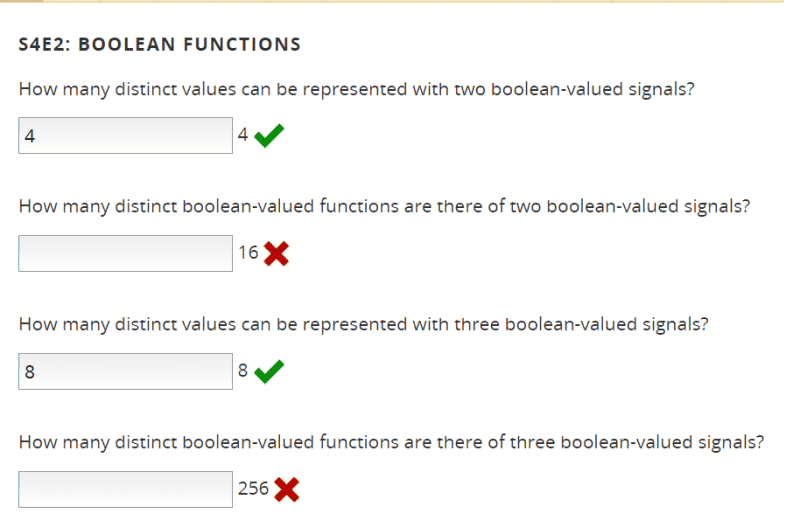# Distinct values represented with boolean-valued signals

• LongApple

## Homework Statement## The Attempt at a Solution

[/B]
I'm trying to parse what the second and fourth questions are asking.
https://en.wikipedia.org/wiki/Boolean-valued_function
I clicked on "preciate" and "proposition" in the wikipedia link and got lost

As a starter, what is an example of a "distinct boolean-valued functions"

and one example of a "two boolean-valued signals" ?

I have a different take than the post on stackexchange, which might or might not be correct. For a boolean-valued function of two boolean signals (second question) there are four distinct arrangements of the two arguments:
f(T, T)
f(T, F)
f(F, T)
f(F, F)
Each of these could result in a function value of either T or F, making a total of 8 different possibilities. For the fourth question, using a similar analysis, I get 16 different possibilities.